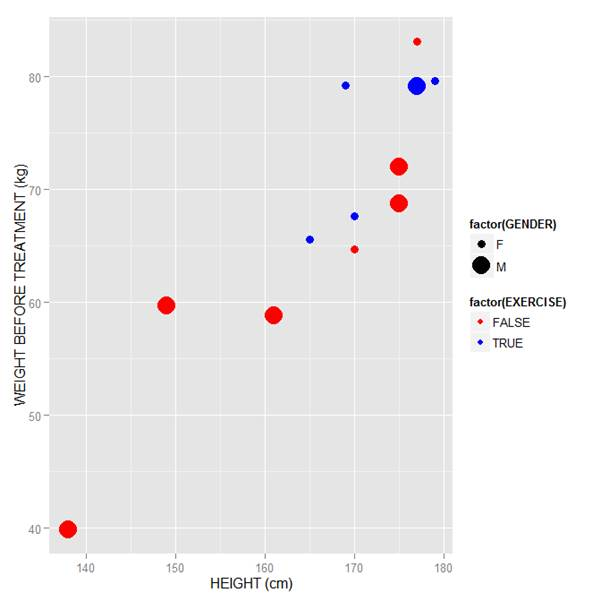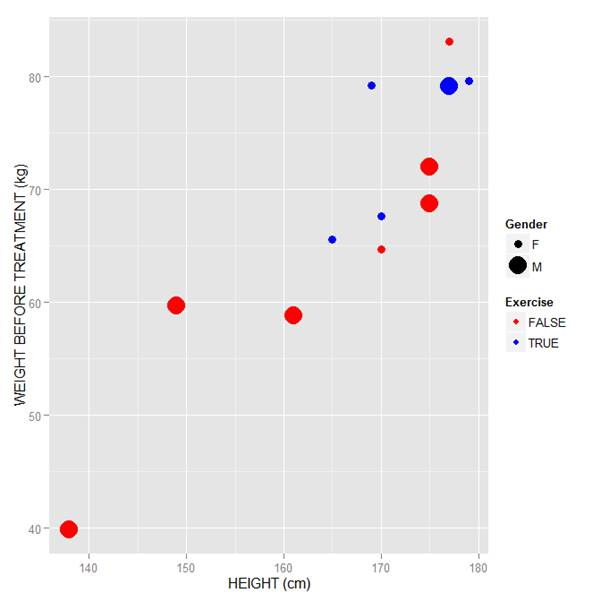# qplot

### R Graphics: Plotting in Color with qplot Part 2

January 13th, 2015 byIn the last lesson, we saw how to use qplot to map symbol colour to a categorical variable. Now we see how to control symbol colours and create legend titles.

```M <- structure(list(PATIENT = c("Mary","Dave","Simon","Steve","Sue","Frida","Magnus","Beth","Peter","Guy","Irina","Liz"), GENDER = c("F","M","M","M","F","F","M","F","M","M","F","F"), TREATMENT = c("A","B","C","A","A","B","A","C","A","C","B","C"), AGE =c("Y","M","M","E","M","M","E","E","M","E","M","M"), WEIGHT_1 = c(79.2,58.8,72.0,59.7,79.6,83.1,68.7,67.6,79.1,39.9,64.7,65.6), WEIGHT_2 = c(76.6,59.3,70.1,57.3,79.8,82.3,66.8,67.4,76.8,41.4,65.3,63.2), HEIGHT = c(169,161,175,149,179,177,175,170,177,138,170,165), SMOKE = c("Y","Y","N","N","N","N","N","N","N","N","N","Y"), EXERCISE = c(TRUE,FALSE,FALSE,FALSE,TRUE,FALSE,FALSE,TRUE,TRUE,FALSE,FALSE,TRUE), RECOVER = c(1,0,1,1,1,0,1,1,1,1,0,1)), .Names = c("PATIENT","GENDER","TREATMENT","AGE","WEIGHT_1","WEIGHT_2","HEIGHT","SMOKE","EXERCISE","RECOVER"), class = "data.frame", row.names = 1:12)```

M

```    PATIENT GENDER TREATMENT AGE WEIGHT_1 WEIGHT_2 HEIGHT SMOKE EXERCISE RECOVER
1     Mary      F         A   Y     79.2     76.6    169     Y     TRUE       1
2     Dave      M         B   M     58.8     59.3    161     Y    FALSE       0
3    Simon      M         C   M     72.0     70.1    175     N    FALSE       1
4    Steve      M         A   E     59.7     57.3    149     N    FALSE       1
5      Sue      F         A   M     79.6     79.8    179     N     TRUE       1
6    Frida      F         B   M     83.1     82.3    177     N    FALSE       0
7   Magnus      M         A   E     68.7     66.8    175     N    FALSE       1
8     Beth      F         C   E     67.6     67.4    170     N     TRUE       1
9    Peter      M         A   M     79.1     76.8    177     N     TRUE       1
10     Guy      M         C   E     39.9     41.4    138     N    FALSE       1
11   Irina      F         B   M     64.7     65.3    170     N    FALSE       0
12     Liz      F         C   M     65.6     63.2    165     Y     TRUE       1
```

Now let’s map symbol size to `GENDER` and symbol colour to `EXERCISE`, but choosing our own colours. To control your symbol colours, use the layer: `scale_colour_manual(values = c())` and select your desired colours. We choose red and blue, and symbol sizes 3 and 7.

`qplot(HEIGHT, WEIGHT_1, data = M, geom = c("point"), xlab = "HEIGHT (cm)", ylab = "WEIGHT BEFORE TREATMENT (kg)" , size = factor(GENDER), color = factor(EXERCISE)) + scale_size_manual(values = c(3, 7)) + scale_colour_manual(values = c("red", "blue"))`

Here is our graph with red and blue points:Now let’s see how to control the legend title (the title that sits directly above the legend). For this example, we control the legend title through the name argument within the two functions `scale_size_manual()` and `scale_colour_manual()`. Enter this syntax in which we choose appropriate legend titles:

```qplot(HEIGHT, WEIGHT_1, data = M, geom = c("point"), xlab = "HEIGHT (cm)", ylab = "WEIGHT BEFORE TREATMENT (kg)" , size = factor(GENDER), color = factor(EXERCISE)) + scale_size_manual(values = c(3, 7), name="Gender") + scale_colour_manual(values = c("red","blue"), name="Exercise") ```We now have our preferred symbol colour and size, and legend titles of our choosing.

That wasn’t so hard! In our next blog post we will learn about plotting regression lines in R.In this lesson, let’s see how to use qplot to map symbol colour to a categorical variable.